Since the development of the Black-Scholes formula for determination of the price of options, the “implied volatility” has been an ordinary tern in markets participants’ vocabulary. Given the non-linear payoff of options, they can be used to implement trading strategies which are not directional (that is, they are not based on a bet on a bullish or bearish view of the underlying), but which profits from changes in the implied volatility. Here, we want to introduce one of this strategy called “Dispersion Trading”, which we will implement in practice in a following article.

Introduction

The Dispersion Trading strategy is based on taking opposite positions on the volatility of an index and its components, which means selling (buying) index options and buying (selling) options on the index components. As an option premium increases with an increase in implied volatility, the mentioned above strategy results in being short (long) index implied volatility and long (short) volatility of the components. In addition, the whole option portfolio is hedged with a position in the underlying stocks, so that it is delta-neutral over the investment horizon.

This strategy aims to profit from the fact that the implied volatility of index options tends to be relatively higher than the theoretical volatility calculated using components’ implied volatilities and betting on the convergence of the two as the options comes to expiry. The common explanation of this phenomenon is the presence of a mispricing in the options markets or of a premium for bearing “correlation risk” in index options.

Researches found that this strategy was systematically profitable, which means it could be simply rolled over after contracts expiration without any market-timing consideration, in the US financial markets before the 2000, after which it does not deliver appealing results. However, according to other publications, profit opportunities rise from time to time and some indicators can be developed to reveal when to enter the position.

Dispersion

The dispersion of an index is a measure of the collective behaviour of its components’ returns around their mean. The more each component’s return deviates from the index’s return (which is the weighted average of the components’ returns), higher the dispersion of the index. This concept is strongly related to correlation: for given volatilities of components, the lower the overall correlation between them, the higher the dispersion of the index (and lower the index volatility). An example is depicted in graph 1: on the left, the components’ returns have high correlation and thus low dispersion, resulting in higher index volatility; on the right, the index is more dispersed, resulting in lower index volatility. Because the market seems to overestimate the index volatility relatively to components volatility, it means that the market is underestimating the index’s dispersion: the markets participants price index options as if the returns were distributed as in the left of graph 1, but in reality, the components behaves like the right side of the graph. The Dispersion Trading strategy requires buying options on components and selling index options, resulting in a long position in the index dispersion.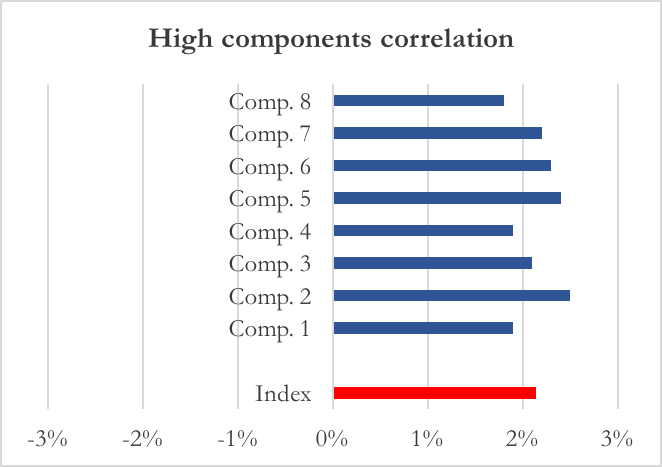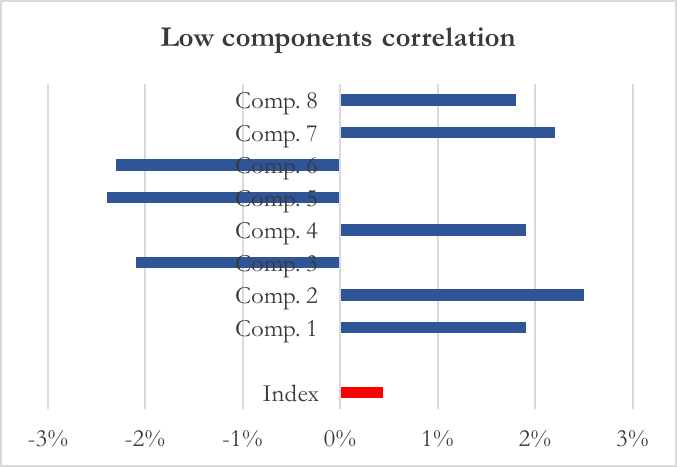Graph 1: index with highly and lowly correlated components

Implied Correlation and Dispersion Indicator

As mentioned earlier, one of the factors that explain a large part of the dispersion of a portfolio or index are the pair-wise correlations between the constituent stocks. However, since most indices consist of numerous stocks, rather than looking at the matrix of pair-wise correlations, it is often more meaningful to find one number that reflects the overall level of correlation.

We know that the volatility of an index or portfolio is given by the formula:Equation 1: σI, σi and σj are respectively the volatilities of the index, the i-th and j-th component, ρij is the correlation between the i-th and j-th components, wi and wj are the weigths of the i-th and j-th components in the index.

In the above formula, instead of taking a matrix of pairwise correlations, we assume one average correlation coefficient, perform some basic algebraic manipulations and get the implied correlation: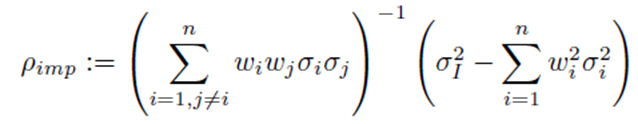Equation 2

Where ρimp represent the average correlation between the index constituents. We could use this implied average correlation as an indicator of the amount of dispersion that the options market is pricing in.

However, dispersion is a result of both correlation and volatility – for example, in Equation 1, a negative correlation’s diversification benefits would be multiplied or amplified by the volatility of i and j. Hence, to get a more holistic picture of dispersion – one that captures the impact of correlation as well as the volatility of the constituents – we use another indicator: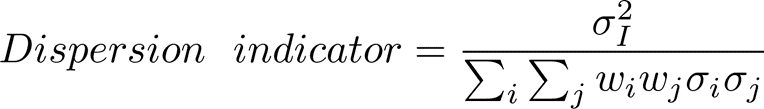Equation 3

This indicator simply divides the implied index volatility by the volatility of a hypothetical portfolio of index constituents with perfectly positive pair-wise correlations. Since the denominator represents the volatility of a portfolio with no dispersion and diversification benefits, the ratio can be interpreted as an inverse measure of the option market’s implied dispersion/risk reduction compared to a situation of zero risk reduction. Lower the dispersion indicator, higher the option-implied dispersion and lower the option-implied correlation.

The Dispersion Indicator acts as a signal to determine entry and exit points for the trade. The entry and exit levels can be determined empirically as key levels at which the indicator generally switches direction or mean-reverts. Suppose we determine 0.8 and 0.4 as two key levels of the dispersion indicator. This would mean that we short the index volatility and go long the constituents’ volatilities every time the indicator is greater than 0.8 (market is pricing in too little dispersion) and square off the trade when it hits 0.4 (market is pricing an acceptable level of dispersion).

Sources of the mispricing

As usual when dealing with phenomena that are not perfectly aligned with the picture of efficient markets, different potential explanations could be hidden behind the dispersion trade’s opportunity.

One of the most frequent explanation employed by market participant claims that since Index options have higher demand than option on single components have, the price of the former is relatively higher than that of the latter. In particular, the majority of the market participants are long-only passive/semi-active funds. These are well-diversified funds that seldom need to buy protection (i.e. buy put options) on individual stocks, which would only partially insure against systematic downside scenarios. Since their portfolios are well diversified, it makes more sense for them to buy protection on diversified indices. Indeed, this is exactly what they do, resulting in the demand for index options being consistently higher than the demand for options on the underlying stocks. Higher demand for index options means higher implied volatilities of index options, other things remaining the same. This means that the implied correlation between the constituents is consistently overstated – since higher implied volatility of the index, implied volatilities of the constituents remaining the same, results in higher implied correlation (remember that volatility of an index/portfolio is positively related to correlation between constituents).

On top of that, Institutional Investors often sell OTM call options on selected single stocks whose performance is expected to be low or flat, so that the option will probably end up OTM. This is a very common practice known as “Call Underwriting”, which allows the seller to cash in the option premium as well as locking in the volatility risk premium embedded in options. As a consequence, the implied volatility of these options decrease, magnifying the mispricing.

Moreover, another factor paving the way to dispersions’ opportunities is the correlation risk premium. The lack of a liquid market for implied correlations requires traders to estimate correlation matrices for pricing multi-asset equity options from historical data. Given the noisy estimation obtained, market participants selling basket options attach a premium to the correlation value used as input for pricing.

Hedging and Weighting strategies

In order for the dispersion trade to be successful, we have to make sure that our position is delta-hedged and as vega sensitive as possible. One way to address the delta-hedging is to enter in straddles or strangles position. Moreover, for what concern the vega exposure, we could enter in several straddles or strangles so that our position does not lose vega as soon as the underlying moves away from the single strike.

The figure below – which represents graphically the same reasoning using single options instead of straddles – should convey the idea. As explained in “More Than You Ever Wanted To Know About Volatility Swaps” (Derman et al., 1999), the best way to obtain an undiluted exposure to variance is indeed through combining options of different strikes – in the limit, when you buy for infinite strikes you obtain a portfolio that is always exposed to variance regardless of the underlying stock price. For the most curious, it turns out that the best way to achieve this result is through weighting the different options for the inverse of the square of the strike in order to balance the increased contribution to vega given by higher strike options. However, this is something that goes beyond the purpose of the dispersion trading strategy.

Once the greeks have been taken care of, we can handle the weighting scheme of the options on single component. One straightforward and very used approach is the vega-weighting approach. The dispersion strategy will be built in a way such that the vega of the index equals the sum of the vegas of the constituents. Therefore, this will immune our trade against short same-direction movements in the volatility of both index and components.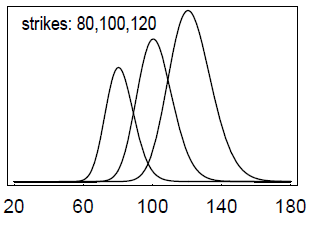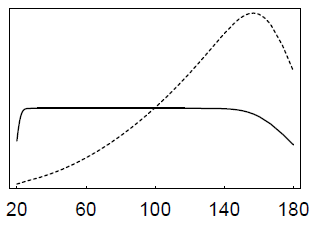Graph 2: on the left side, vega of single options with different strikes; on the right, vega of a portfolio of options with different strikes (Source: “More Than You Ever Wanted To Know About Volatility Swaps” (Derman et al., 1999)

Stay tuned: the in depth implementation of the strategy is about to come in the next newsletter

Categories: Markets